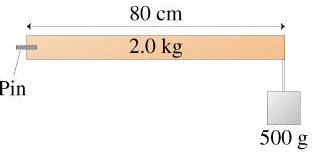# Problem: How much torque must the pin exert to keep the rod in the figure from rotating? Calculate this torque about an axis that passes through the point where the pin enters the rod and is perpendicular to the plane of the figure.

###### FREE Expert Solution

The 500 g weight provides a clockwise torque 80 cm from the axis of rotation.

The weight of the rod provides a clockwise torque, 40 cm from the axis of rotation.

The torque exerted by the pin should be in the opposite direction (counterclockwise).

Weight of 500 g mass = mg = 500 g (1 kg / 1000 g) × 9.8 m/s2 = 4.9 N###### Problem Details

How much torque must the pin exert to keep the rod in the figure from rotating? Calculate this torque about an axis that passes through the point where the pin enters the rod and is perpendicular to the plane of the figure.